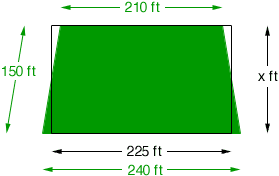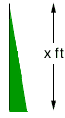Quandaries and Queries I have a lot that is 210 feet in the front, 240 feet in the back and the sides each measure 150 feet. How many square feet is this all together and how close to an acre is it? I'm a senior (12th ). Linda Hi Linda, I drew an approximately scale drawing of your lot, in green. If you take the average of 210 feet and 240 feet you get 225 feet and you can see that the black rectangle I have drawn with one side 225 feet has the same area as your lot.What is unknown is the other dimension of the rectangle, which I have labeled x feet. (It will be almost 150 feet, just slightly shorter.) If you move the right, black line 15 feet to the left you form a right triangle with height x ft, base 30 ft and hypotenuse 150 ft.Usinf the theorem of Pythagoras, x2 + 302 = 1502. Solving for x I get x = 147 ft. Thus your lot has an area which is the same as the area of a rectangle, 225 ft by 147 ft. Hence the area is 225147 = 33,075 square feet. An acre is 43560 square feet and hence your lot is  33075/43560 = 0.76 of an acre. That is about three-quarters of an acre. Cheers, Penny Go to Math Central